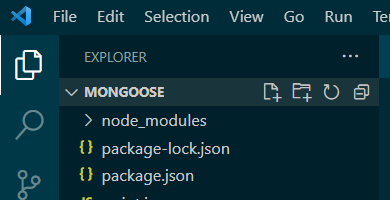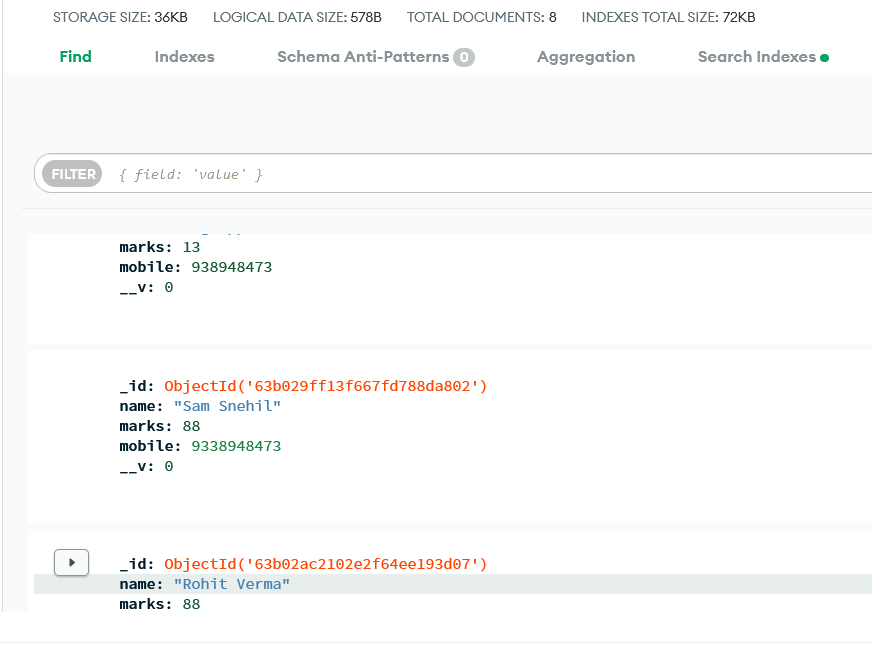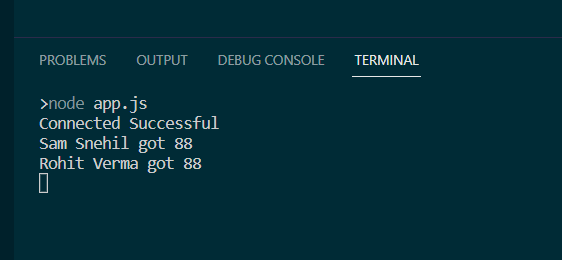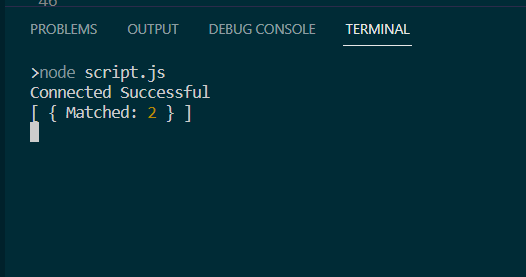GeeksforGeeks App
Open AppBrowser
Continue

# Mongoose prototype.Aggregate() API

The API.prototype.Aggregate() method of the Mongoose API is used to build aggregation pipelines. Aggregation is a method of processing many documents by sending them through multiple phases.

Syntax:

`Model.aggregate()`

Parameters:

• pipeline: It is an array that is an aggregation pipeline as an array of objects.
• model: It is a method to use with this aggregate.

Returns: It returns plan JavaScript Objects.

Setting up Node.js application:

Step 1: Create a Node.js application using the following command:

`npm init`

Step 2: After creating the NodeJS application, Install the required module using the following command:

`npm install mongoose`

Project Structure: The project structure will look like this:output

Example 1: In this example, we have established a database connection using mongoose and defined a model over schema, having three columns or fields “name”, “marks” and “mobile”. In the end, we are using the aggregate() method on the User model which will use match and filter some tuples of lists from the User collection.

Database Structure: The database structure will look like this, Three documents are present in the collection over which we match the document with marks greater than 87. In collection, we have only two documents with marks greater than 87.output2

Filename: app.js

## Javascript

 `// Require the mongoose module``const mongoose = require(``"mongoose"``);` `// Path to our cloud DataBase``const url = ``"mongodb://localhost:27017/geeksforgeeks"``;` `// Connecting to database``mongoose.set(``"strictQuery"``, ``false``);` `mongoose``    ``.connect(url)``    ``.then((ans) => {``        ``console.log(``"Connected Successful"``);``    ``})``    ``.``catch``((err) => {``        ``console.log(``"Error in the Connection"``);``    ``});` `// Calling Schema class``let Schema = mongoose.Schema;` `// Creating Structure of the model``let schema = ``new` `Schema({``    ``name: String,``    ``marks: Number,``    ``mobile: Number,``});``// compile our model``let Person = mongoose.model(``"gfgs"``, schema);` `let find = async () => {``    ``const aggregate = await Person.aggregate([``        ``{ \$match: { marks: { \$gte: 87 } } },``    ``]);` `    ``return` `aggregate;``};` `find().then((x, y) => {``    ``for` `(let i of x) console.log(`\${i.name} got \${i.marks}`);``});`

Output:output3

Example 2: In this example, we are using the count pipeline inside the asynchronous function to count the matched document inside the collection.

Filename: script.js

## Javascript

 `// Require the mongoose module``const mongoose = require(``'mongoose'``);` `// Path to our cloud Database``const url = ``'mongodb://localhost:27017/geeksforgeeks'` `// Connecting to database``mongoose.set(``'strictQuery'``, ``false``);` `mongoose.connect(url)``    ``.then((ans) => {``        ``console.log(``"Connected Successful"``)``    ``})``    ``.``catch``((err) => {``        ``console.log(``"Error in the Connection"``)``    ``})` `// Calling Schema class``const Schema = mongoose.Schema;` `// Creating Structure of the model``let schema = ``new` `Schema({``    ``firstName: String,``    ``lastName: String,``});` `// Compile our model``let Person = mongoose.model(``'person'``, schema);` `let find = async () => {``    ``const aggregate = await Person.aggregate([``        ``{ \$match: { lastName: ``'Verma'` `} },``        ``{ \$count: ``'Matched'` `}``    ``]).exec((err, fi) => {``        ``if` `(err) ``throw` `err;``        ``console.log(fi);``    ``})``    ``return` `aggregate;``}` `find();`

Output:output4

My Personal Notes arrow_drop_up
Related Tutorials